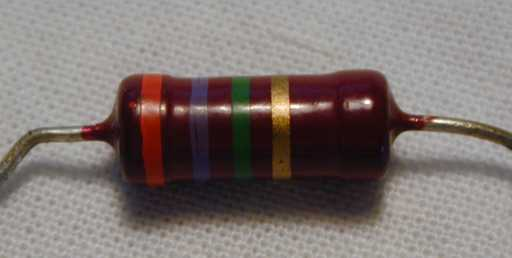Resistor are designed to Resist current. That is they limit flow. They are one of the most common electronic components you will come across.

They come in a few forms - the most common being a small cylinder in shades of brown or blue with a set of coloured bands and two wires sticking out.You may find some in larger sealed packages with the value printed on them.

Another form are resister networks/packs - a SIL or DIL chip - with many resistors on it, often with the same value- and sometimes sharing a common connection. Resistor packs are useful if you are going to use many resistors of the same value.

You will note there are two symbols for resistors. I tend to use the simpler of the two as it is easier to draw, although the latter is more distinctive. Whichever convention you use - try to stick to it, at least use the convention in one diagram!

I, like many others, like to use water pipe analogies to explain electronic components. Imagine a pipe that is thinner than others - less water will flow through the pipe, although the water flow rate will be faster.

Resistance in electronics is usually represented by the equation V=IR - that is, the voltage (V) across two points is equal to its resistance (R), multiplied by the current (R). This can be written in other forms - I=V/R - so if you increase the resistance, on a constant voltage device, then the current will decrease. Back to the water pipe - this means voltage is the flow rate, and the current is the flow amount.

## Resistor Colour Bands

</table>
Colour BandNumeric ValueMultiplierTolerance
Black01
Brown 1101%
Red21002%
Orange31000(1k)
Yellow410k
Green5100k
Blue61M
Violet710M
Gray8100M
White9
Gold 0.15%
Silver 0.0110%